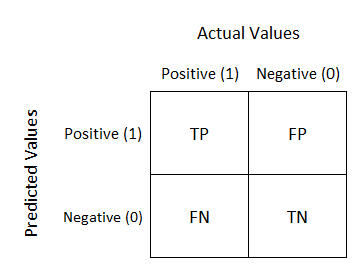2 views

I have a dataset which is a large JSON file. I read it and store it in the trainList variable.

Next, I pre-process it - in order to be able to work with it.

Once I have done that I start the classification:

1. I use the kfold cross-validation method in order to obtain the mean accuracy and train a classifier.

2. I make the predictions and obtain the accuracy & confusion matrix of that fold.

3. After this, I would like to obtain the True Positive(TP), True Negative(TN), False Positive(FP) and False Negative(FN) values. I'll use these parameters to obtain the Sensitivity and Specificity.

Finally, I would use this to put in HTML in order to show a chart with the TPs of each label.

Code:

#The variables I have for the moment:

trainList

#I transform the data from JSON form to a numerical one X=vec.fit_transform(trainList)

#I scale the matrix (don't know why but without it, it makes an error)

X=preprocessing.scale(X.toarray())

#I generate a KFold in order to make cross validation

kf = KFold(len(X), n_folds=10, indices=True, shuffle=True, random_state=1)

#I start the cross validation

for train_indices, test_indices in kf:

X_train=[X[ii] for ii in train_indices]

X_test=[X[ii] for ii in test_indices]

y_train=[listaLabels[ii] for ii in train_indices]

y_test=[listaLabels[ii] for ii in test_indices]

#I train the classifier trained=qda.fit(X_train,y_train)

#I make the predictions predicted=qda.predict(X_test)

ac=accuracy_score(predicted,y_test)

#I obtain the confusion matrix

cm=confusion_matrix(y_test, predicted)

#I should calculate the TP,TN, FP and FN

#I don't know how to continue

by (33.1k points)

You can obtain True Positive (TP), True Negative (TN), False Positive (FP) and False Negative (FN) by implementing confusion matrix in Scikit-learn.

Confusion Matrix:

It is a performance measurement for machine learning classification problem where output can be two or more classes. It is a table with 4 different combinations of predicted and actual values.True Positive:

Interpretation: You predicted positive and it’s true.

True Negative:

Interpretation: You predicted negative and it’s true.

False Positive: (Type 1 Error)

Interpretation: You predicted positive and it’s false.

False Negative: (Type 2 Error)

Interpretation: You predicted negative and it’s false.

For example:

> from sklearn.metrics import confusion_matrix

> y_true = [2, 0, 2, 2, 0, 1]

> y_pred = [0, 0, 2, 2, 0, 2]

> confusion_matrix(y_true, y_pred)

array([[2, 0, 0],

[0, 0, 1],

[1, 0, 2]])

> tn, fp, fn, tp = confusion_matrix([0, 1, 0, 1], [1, 1, 1, 0]).ravel()

> (tn, fp, fn, tp)

(0, 2, 1, 1)

Hope this solution helps.